# 覺得 JavaScript function 很有趣的我是不是很奇怪

## 前言

### 問題一：Named function expression

var fib = function(n) {
if (n <= 1) return n
return fib(n-1) + fib(n-2)
}

console.log(fib(6))


var fib = function calculateFib(n) {
if (n <= 1) return n
return fib(n-1) + fib(n-2)
}

console.log(calculateFib) // ???
console.log(fib(6))


1. 那這個 function 到底叫做 fib 還是 calculateFib？
2. 底下那行 console.log(calculateFib) 會輸出什麼？
3. 既然前面都已經給它名字了，為什麼後面還要再多一個？

### 問題二：apply 與 call

1. 直接呼叫
2. call
3. apply

function add(a, b) {
console.log(a+b)
}



1. 為什麼除了一般的呼叫 function 以外，還需要 call 跟 apply？什麼情形下需要用到它們？

### 問題三：建立函式

1. Function declaration
2. Function expression
3. Function constructor

// Function declaration
function a1(str) {
console.log(str)
}

// Function expression
var a2 = function(str) {
console.log(str)
}

// 很少看到的 Function constructor
var a3 = new Function('str', 'console.log(str)')

a1('hi')
a2('hello')
a3('world')


var obj = {
hello() {
console.log(1)
}
}

obj.hello()


### 問題四：黑魔法

function log(obj) {
console.log(obj.str)
}

log({str: 'hello'})


function log(obj) {
doSomeMagic()
console.log(obj.str) // 要讓這邊輸出的變成 world
}

// 只能改動這個函式裡面的東西
function doSomeMagic() {
// 在這邊施展魔法
}

log({str: 'hello'})


## Fun fun function

function add(a, b) {
return a + b
}

// 正常呼叫

// 當成一般物件

// 當成陣列

for(let i=0; i<5; i++) {
}


function add(a, b) {
return a + b
}

// 當成陣列



function add(a, b) {
console.log(arguments) // [Arguments] { '0': 1, '1': 2 }
console.log(arguments.length) // 2

// 像陣列一樣操作
for(let i=0; i<arguments.length; i++) {
console.log(arguments[i])
}

// 可是不是陣列
console.log(Array.isArray(arguments)) // false
console.log(arguments.map) // undefined
}



function add(a, b) {
let a1 = Array.from(arguments)
console.log(Array.isArray(a1)) // true
}


function add(a, b) {
let a2 = Array.prototype.slice.call(arguments)
console.log(Array.isArray(a2)) // true
}


console.log([].slice === Array.prototype.slice) // true


// 提醒一下，幫不屬於自己的物件加上 prototype 不是一件好事
// 應該盡可能避免
Array.prototype.first = function() {
return this
}

console.log(.first()) // 1
console.log([2,3,4].first()) // 2


22.1.3.25 Array.prototype.slice 可以看到相關說明跟運行方式，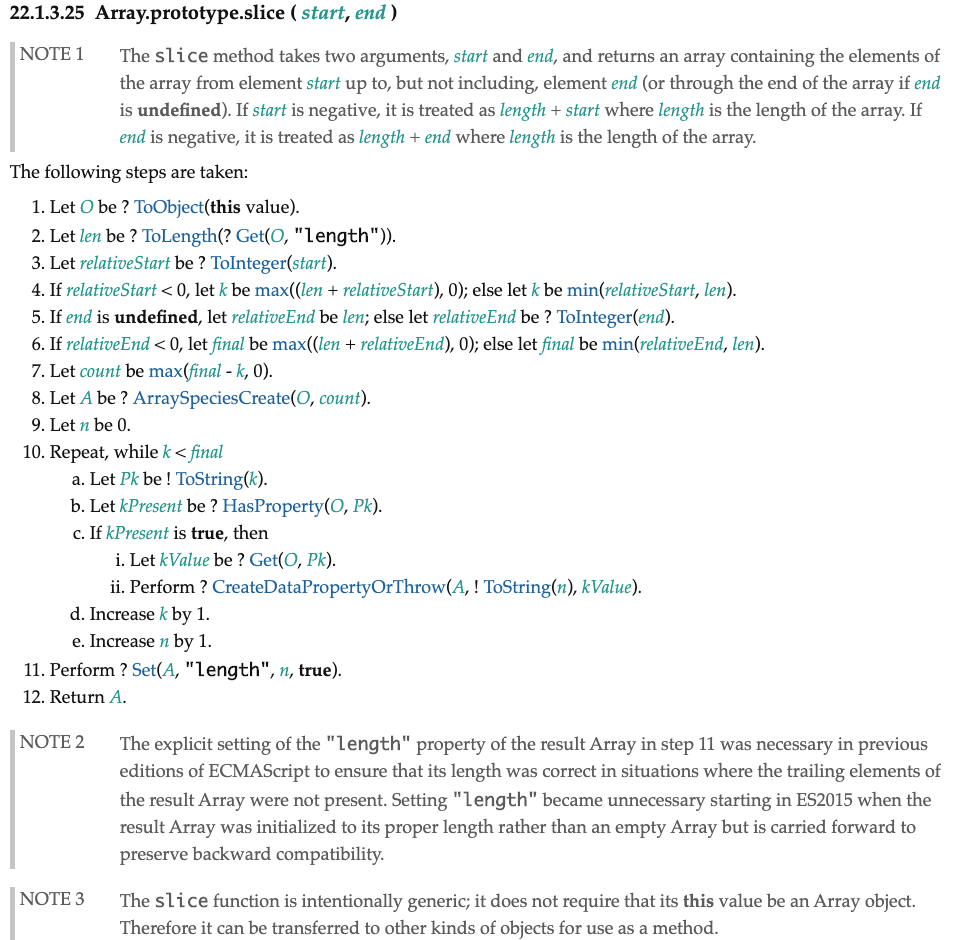The slice function is intentionally generic; it does not require that its this value be an Array object. Therefore it can be transferred to other kinds of objects for use as a method.

// 記得這邊參數一定要是三個，才能讓長度變成 3
function test(a,b,c) {}
test = 1
test = 2
test = 3

// function 搖身一變成為陣列
var arr = Array.prototype.slice.call(test)
console.log(arr) // [1, 2, 3]


console.log(Math.max(1,2,3,4,5,6)) // 6


var arr = [1,2,3,4]
console.log(Math.max(arr)) // NaN


var arr = [1,2,3,4]
console.log(Math.max.apply(null, arr)) // 4


var arr = [1,2,3,4]
console.log(Math.max(...arr)) // 4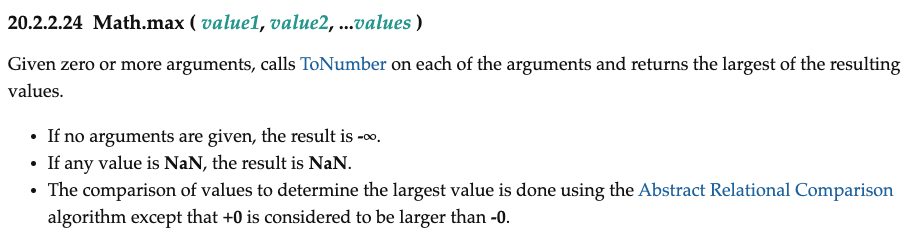var arr = []
var obj = {}
var fn = function(){}
console.log(arr.toString()) // 空字串
console.log(obj.toString()) // [object Object]
console.log(fn.toString()) // function(){}


function isObject(obj) {
if (!obj || !obj.toString) return false
return obj.toString() === '[object Object]'
}

var arr = []
var obj = {}
var fn = function(){}
console.log(isObject(arr)) // false
console.log(isObject(obj)) // true
console.log(isObject(fn)) // false


function isObject(obj) {
if (!obj || !obj.toString) return false
return obj.toString() === '[object Object]'
}

var obj = {
toString: function() {
return 'I am object QQ'
}
}

console.log(isObject(obj)) // false


function isObject(obj) {
if (!obj || !obj.toString) return false

// 新的
return Object.prototype.toString.call(obj) === '[object Object]'

// 舊的
// return obj.toString() === '[object Object]'
}

var obj = {
toString: function() {
return 'I am object QQ'
}
}

console.log(isObject(obj)) // true


（附註：以上判斷物件的方法應該還是有一些 case 過不了，但我只是想示範 call 的存在理由之一，並不是真的想寫出 isObject 這個函式）

## 神秘的 function 自帶變數

function test(a) {
console.log(a) // 1
console.log(arguments) // 1
a = 2
console.log(a) // 2
console.log(arguments) // 2
arguments = 3
console.log(a) // 3
console.log(arguments) // 3
}
test(1)


function log(obj) {
doSomeMagic()
console.log(obj.str) // 要讓這邊輸出的變成 world
}

// 只能改動這個函式裡面的東西
function doSomeMagic() {
// 在這邊施展魔法
}

log({str: 'hello'})


function log(obj) {
doSomeMagic()
console.log(obj.str) // 要讓這邊輸出的變成 world
}

// 只能改動這個函式裡面的東西
function doSomeMagic() {
// magic!
log.arguments.str = 'world'
}

log({str: 'hello'})


(function(obj) {
doSomeMagic()
console.log(obj.str) // 要讓這邊輸出的變成 world
})({str: 'hello'})

// 只能改動這個函式裡面的東西
function doSomeMagic() {

}


The function.caller property returns the function that invoked the specified function. It returns null for strict, async function and generator function callers.

function a(){
b()
}

function b(){
console.log(b.caller) // [Function: a]
}

a()


(function(obj) {
doSomeMagic()
console.log(obj.str) // 要讓這邊輸出的變成 world
})({str: 'hello'})

// 只能改動這個函式裡面的東西
function doSomeMagic() {
doSomeMagic.caller.arguments.str = 'world'
}


(function() {
(function() {
// 只能改動這個函式

})()
console.log(arguments.str) // 要讓這邊輸出的變成 world
})({str: 'hello'})


## 建立函式

1. Function declaration
2. Function expression
3. Function constructor

var f = new Function('str', 'console.log(str)')
f(123)


var f = Function.constructor('str', 'console.log(str)')
f(123)


var f = Function('str', 'console.log(str)')
f(123)


function Dog(name) {
this.name = name
}

Dog.prototype.sayHi = function() {
console.log('I am', this.name)
}

let d = new Dog('yo')
d.sayHi() // I am yo


function Dog(name) {
this.name = name
}

Dog.prototype.sayHi = function() {
console.log('I am', this.name)
}

let d = new Dog('yo')
d.sayHi() // I am yo

console.log(d.constructor) // [Function: Dog]


function test() {}

console.log(test.constructor) // [Function: Function]
console.log(test.constructor === Function.constructor) // true


function test() {}

var f = test.constructor('console.log(123)')
f() // 123


var f1 = [].map.constructor('console.log(123)')
var f2 = Math.min.constructor('console.log(456)')
f1() // 123
f2() // 456


// function declaration
function a() {}

// function expression
var b = function() {}


// function declaration
a()
function a() { }


// function expression
b() // TypeError: b is not a function
var b = function () {}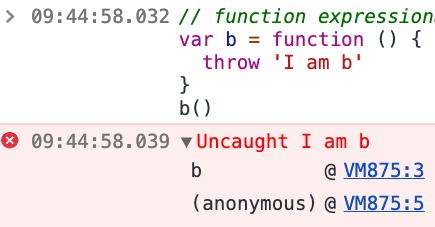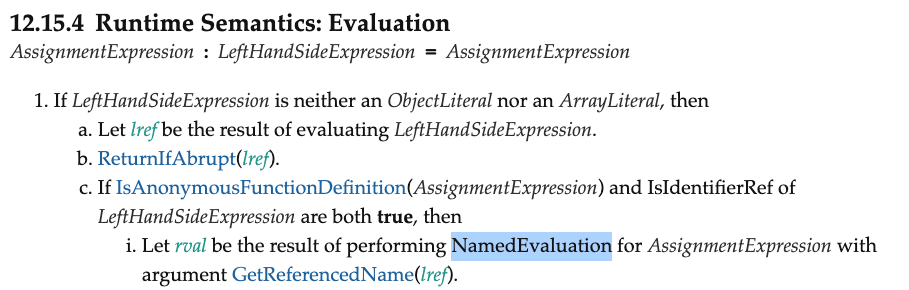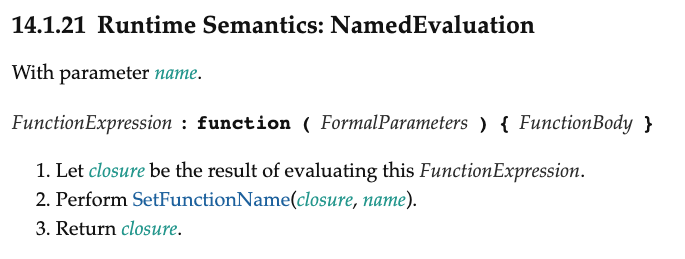// function expression
var b = function helloB() {
throw 'I am b'
}
b()


// function expression
var b = function helloB() {
throw 'I am b'
}
helloB() // ReferenceError: helloB is not defined


// function expression
var b = function fib(n) {
if (n <= 1) return n
return fib(n-1) + fib(n-2)
}
console.log(b(6)) // 8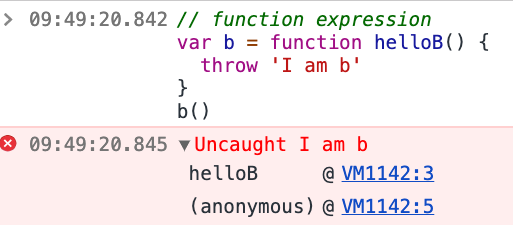var arr = [1,2,3,4,5]
var str =
arr.map(function(n){ return n + 1})
.filter(function(n){ return n % 2 === 1})
.join(',')
console.log(str) // 3, 5


var arr = [1,2,3,4,5]
var str =
arr.map(function(n){ return n + 1})
.filter(function(n){ throw 'errr' })
.join(',')
console.log(str) // 3, 5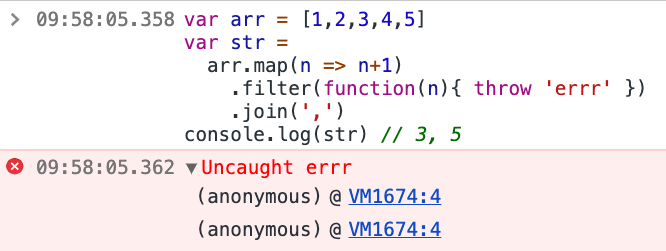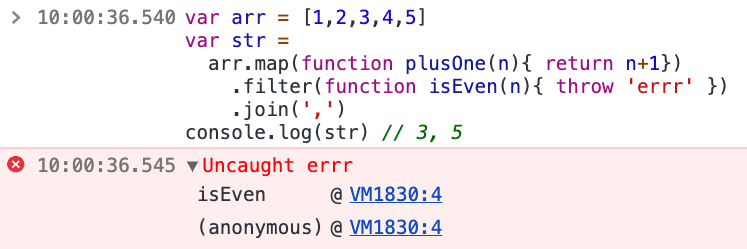function run(fn, n) {
console.log(fn(n)) // 55
}

run(function fib(n) {
if (n <= 1) return n
return fib(n-1) + fib(n-2)
}, 10)


run 只是一個空殼，會接收一個函式跟一個參數，接著就只是呼叫函式然後把執行結果印出來。在這邊我們傳入一個 named function expression 來算費氏數列，因為需要遞迴的關係，所以才幫函式取了名稱。

function run(fn, n) {
console.log(fn(n)) // 55
}

run(function (n) {
if (n <= 1) return n
return arguments.callee(n-1) + arguments.callee(n-2)
}, 10)


arguments 這個神奇的物件前面已經介紹過了，但沒有講到的是上面有一個屬性叫做 calleeMDN 的解釋是：

callee is a property of the arguments object. It can be used to refer to the currently executing function inside the function body of that function. This is useful when the name of the function is unknown, such as within a function expression with no name (also called "anonymous functions").

(function() {
(function() {
// 只能改動這個函式

})()
console.log(arguments.str) // 要讓這邊輸出的變成 world
})({str: 'hello'})


(function() {
(function() {
// 只能改動這個函式
arguments.callee.caller.arguments.str = 'world'
})()
console.log(arguments.str) // 要讓這邊輸出的變成 world
})({str: 'hello'})


## 解答時間

var fib = function calculateFib(n) {
if (n <= 1) return n
return fib(n-1) + fib(n-2)
}

console.log(calculateFib) // ???
console.log(fib(6))


### 2. 底下那行 console.log(calculateFib) 會輸出什麼？

ReferenceError: calculateFib is not defined

### 3. 既然前面都已經給它名字了，為什麼後面還要再多一個？

1. 想呼叫自己的時候可以用這個名稱
2. stacktrace 會出現這個名字

### 4. 為什麼除了一般的呼叫 function 以外，還需要 call 跟 apply？什麼情形下需要用到它們？

1. 當我們想傳入陣列，但原本的函式只支援一個一個參數的時候
2. 當我們想自訂 this 的時候
3. 當我們想避開函式覆寫，直接呼叫某個函式的時候

### 5. 有沒有辦法做到不用 function 關鍵字，也能建立函式呢？

var f1 = [].map.constructor('console.log(123)')
var f2 = Math.min.constructor('console.log(456)')
f1() // 123
f2() // 456